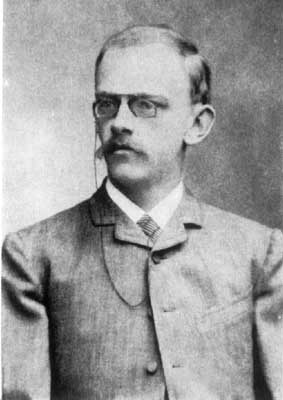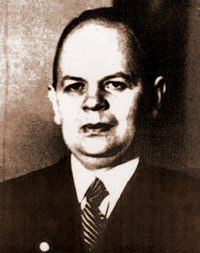# bigΨ.I.Number operations

## Ψ.2.Up to the Ackermann numbers

#### §2.0.1.Star spangled superpowersAllahu Akbar !

God is greater

– Call to prayer, Islam

Exponentiation `a^b` or raising to a power is the operation which iterates over multiplication. In this chapter the double star `**` rather than the arrowhead `^` functions as the exponential operator.
The hardly extendible superscript notation ab is regarded as a remnant of a past, now gone.

a**0 = 1
a**b = a*... {a#b} `= a^b`

Next comes the true superpower with three stars `***` which Goodstein called tetration (tetra = 4) counting index `1` for addition (here `0` stars).
Our three stars equals two `^^` arrowheads in Donald Knuth's 1976 up-arrow notation – where the operand `b` always counts repetitions of items `a`. And not to Ackermann's phi recursion – because Ackermann's own iterator `b` counts the number of in-between arrow operators instead.

a***0 = 1
a***b = a**... {a#b} `= a^^b`

The insight that `*` is a countable unit, dawns with the recognition that operators `*..` can be expanded at will. Of the various names for this family of operations that have been suggested, Nick Bromer's superexponentiation is most instructive. But Bromer's term is verbally overweight, we'll use our superpowers instead.

In David Hilbert's "On the infinite" a functional (function that takes a function as an argument) is deemed necessary to define this, while it follows immediately from Hilbert's own formula, that an ordinary function does the trick. Perhaps Hilbert didn't like variables of a next recursive level `n1` substituted as arguments in lower type `n` functions, but his roundabout definition cannot hide the fact that this follows immediately by `=`.

• F0(a,b) = ab
• Fn1(a,1) = I(Fn,a,1) = a
• Fn1(a,b1) = I(Fn,a,b1)
= Fn(a,I(Fn,a,b))
= Fn(a,Fn1(a,b)) == Fn(a,..a.) {#b#}

Note that superpower star operations aren't really built upon powers, because exponentiation `a**b` is the 2nd true operation in the list. The initial operation `ab` of zero stars, which is empty and naturally resolved as addition, forms the start at c=0 of the superpower series, where `c` counts the number of stars and c=1 is multiplication.

From the initial superpower rule `a*1 = a` the next operations `*..` are recursively `==` defined, so that every reduction train stops at a well defined initial case.
Follows the general superpower formula providing for an arbitrary number `c` of stars.

• a*...1 {*#c1} = a*...1 {*#c c>0} == a*1 = a
• a*...11 {*#c1} = a*...a {*#c}   so 11*..11 == 1111
• a*...b1 {*#c1} = a*...(a*...b) {*#c *#c1}
== a*.. ... {*#c a#b1}

Find instructions for calculating with numbers of superpower size in the subchapter on super-calculation.

##### Evaluation of `*` and `^` operators

An `n1` number of superstars in `a*...b` equals `n` arrows (or wedges) in `a^..b`
But there are differences with respect to their order` ` of evaluation or precedence in complex expressions. Given competing star operations `a*..b*..c` (without brackets) we evaluate the smaller operation with less stars before the larger operation. This we call minority precedence,` ` contrary to the common majority precedence, which applies to arrow operations `a^..b^..c`
We let arrows take precedence above stars,` ` so in expressions where both stars and arrows appear, every arrow operation is resolved first.` ` Types of precedence are further investigated in subchapter 2.5.

#### §2.0.2.Primitive recursive functionsDavid Hilbert
(1862-1943)

We'll first explain what recursion is and what primitive recursion,` ` and how all superpowers `a*..b` can be created just by primitive recursion. This answers to the meta-mathematical need to classify` ` a function according to the simplest means with which` ` to generate it.

Recursion is an initial case plus an iterative function which lets all next steps `n1` follow from current step `n`.
Primitive recursion (p.r.) initially increments a parameter by `1` as in `F0(n) = n1`.
All next steps `n1` of functions `Fc1(R,n1)` can only be defined by substitution of a parameter in a known p.r. function `Gc(X)` for a previously defined step `Fc1(R,n)`.
The enumeration of p.r. levels is not made iterable by setting up an extra parameter `c` to expand the levels of the function family `F` directly. Therefore the primitive recursive class of functions remains

As an example we'll generate a primitive recursive function family` ` `Fac` which is a bit special because we start with a function index -1 where 0 is more common.` ` `Fa` is faster than star superpowers, by `Fac(a,0)` `= a` instead of `a*..0 = 1` {*#c c>1} so that for example` ` `Fa2(a,1)` = a+a×a

The inverse unit can signify both the negative index `- = -1` and subtraction as in `a-` = a-1. The applied list markers are explained in section 4.0.5.
Family `Fac` could very well have used one rule `Fa0(a,b) = ab` for initialization. Then only two axioms would have sufficed to define `Fa` instead, which is one less than for superstar or arrow operations.

• Fa-(a,0) = 1
• Fa-(a,b) = Fa-(a,b-)1 == 1... {#b1} = b1
• Fac(a,0) {c≥0} = a
• Fa0(a,1) = Fa-(a,Fa0(a,0)) = Fa-(a,a) = a1
• Fa0(a,b) = Fa-(a,Fa0(a,b-)) == Fa-(a,..a.) {#b#} = ab
• Fa1(a,b) = Fa0(a,Fa1(a,b-)) == Fa0(a,..a.) {#b#} = a*b1
• Fa2(a,b) == Fa1(a,..a.) {#b#} = (a**i)... {#b1 0*}
• Fac(a,b) = Fac-(a,Fac(a,b-)) > a*...b1 {*#c a>0 b>0 c>1}

The reason why `Fa` didn't make it as a defining algorithm for superpowers is that its properties are not so cool as those of multiplication, etc… Because `a*b = b*a` is commutative and `Fa1(a1,b)` `= Fa1(b1,a)` is not.
And although the virtues of exponentiation are distributive, with a minor adaptation using logarithms the powers can be rendered commutative with `a**(log(b)*e/log(e))` where `e` is an arbitrary exponent or base.

#### §2.0.3.Ackermann's jumpWilhelm Ackermann
(1896-1962)

Ackermann used a primitive recursive φ function (phi) as a prerequisite to construct the higher level ψ function in his 1928 article "On Hilbert's construction of the real numbers".
The `Ack_ψ` (ψ) function makes the jump from primitive recursion into the vast unknown.

The `Ack_φ` (φ) function is a superpower algorithm, it differs from star operations only with respect to the initial cases for operators c>2. After that it grows away slightly from superpowers, though `Ack_φ` never catches up with the upstart function family `Fac` which raised its initial case earlier at `Fa1(a,0) = a`

• Ack_φ(a,b,0) = ab = a+b
• Ack_φ(a,0,1) = 0
• Ack_φ(a,0,2) = 1
• Ack_φ(a,0,c) {c>11} = a (Ackermann's extra axiom)
• e.g. Ack_φ(a,1,11) = a
•      Ack_φ(a,b,11) = a**b = a^b
•      Ack_φ(a,1,111) = a**a
•      Ack_φ(a,b,111) = a***b1 = a^^(b+1)
• Ack_φ(a,b1,c1) = Ack_φ(a,Ack_φ(a,b,c1),c)

If you'd replace the 2nd and 3d rule by a single axiom `Ack_φ(a,1,c)` {0<c<3} `= a` the evaluation of all expressions of `Ack_φ` where b>0 will work out fine.

Compare the relative speed of the functions of superpowers from this chapter. The three of them are relatively close, with differing values of `b`, while arrows `a^...b {^#c}` increase a unit `1` of `c` faster than stars.

• a*...b {*#c c>11} < Ack_φ(a,b,c) < Fac(a,b) {a>0 b>0}

We still owe you Ackermann's historical psi-function which gave rise to the concept of the Ackermann numbers. The whole Shakespearean purpose of Ackermann's `Ack_ψ` function was not to be primitive recursive, which succeeded gloriously. Yet there was no mention of any Big numbers in Ackermann's famous article.

• Ack_ψ(a) = Ack_φ(a,a,a)
• Ack_ψ(i) == { 1, 4, 3^7625597484987,
4^^(4^^(4^^(4^^5+1)+1)+1), .. }
• ψ(4) = φ(4,4,4) = φ(4,φ(4,3,4),3) = φ(4,φ(4,φ(4,2,4),3),3)
= φ(4,φ(4,φ(4,φ(4,4,3),3),3),3)
where  φ(a,b,3) = φ(a,..φ(a,1,3).,2) {#b-#}
= φ(a,..a.,2) {#b#}
= a**... {a#b1} = a***b1 = a^^(b+1)

The complications in working out the structure of the 4th Ackermann number seems to show that his extra axiom is apart from being unnecessary also relatively unnatural. But this depends on our view of naturalness, which is tainted by Donald Knuth's up-arrow type of superpowers. How cumbersome we may feel about defining extra axioms is not a strong argument in this discussion, given the leaner physique of `Fa` with its meaner power operation `Fa2`, which is similar to the function `Ack_φ(a,b,4)` and just as unwieldy if translated to an expression with stars/arrows.
Anyhow, without the extra axiom the resulting φ' function has the same rules as the superpower stars of bigI. And an expression `Ack_ψ'(4)` reduces to `4^^^4` `= 4^^4^^4^^4` straightforwardly (in our eyes ;-)

#### #Big function classes

Initially all of arithmetic boils down to addition and the natural numbers of Peano's successor function `S(n)` `= n1` which share the same ground level `{K.0}` of complexity – the number class.

a(b1) = 1.. {1#ab1} = (ab)1  `{K.0.ab}` = a+b+1

Surely appending `1` is simpler than adding `b1`, but counting from `1..` {1#ab} is more complex than having `a` in the first place. And philosophically, who says that anything happens in the construction of a number? Any evaluation is essentially a series of notational reflections on equivalent number states.
For example exponential class `{K.1.1}` functions are created by repeating class `{K.1.0}` multiplications. But in writing `a*... {a#b}` for `a**b` it looks like one form of complexity is exchanged for another.

All functions up to our class `{K.1.1}` are called elementary functions. The superpowers dwell on the level `{K.1}` of primitive recursion, where a function `{K.1.c1}` is composed of repeated substitutions (nesting) of earlier primitive recursive functions `{K.1.c}` and its subclass counter `c1` enumerates an equivalent number of star `*..` {*#c2} operators. For a long time mathematicians could see no further.
But in 1928 Wilhelm Ackermann proved a conjecture by Hilbert that there are recursions which increase faster than any primitive recursive function. These are the so called Ackermann functions `{K.2.0}` which iterate over the enumeration coefficient `c` of primitive recursive functions. For example the famous Graham's number is best expressed by an early class `{K.2.0}` function.

As iteration of repetitions assigns a subclass `{K.1.c}` to a superpower, so can the number of subsequent Ackermann jumps in a higher function be used to enumerate its subclass `{K.2.d}`.
In chapter 5 we'll prove that each of the chained arrows advanced by John H. Conway exactly represents such an Ackermann jump, and their first row covers the level `{K.2}` of what we've dubbed Ackermann-Conway functions. Where the length of a row of chained arrows can be expressed by the value of parameter `d` in bigE (or by the fourth entry in Bowers' Exploding Array Function) this gives us a model of the subclass counter.